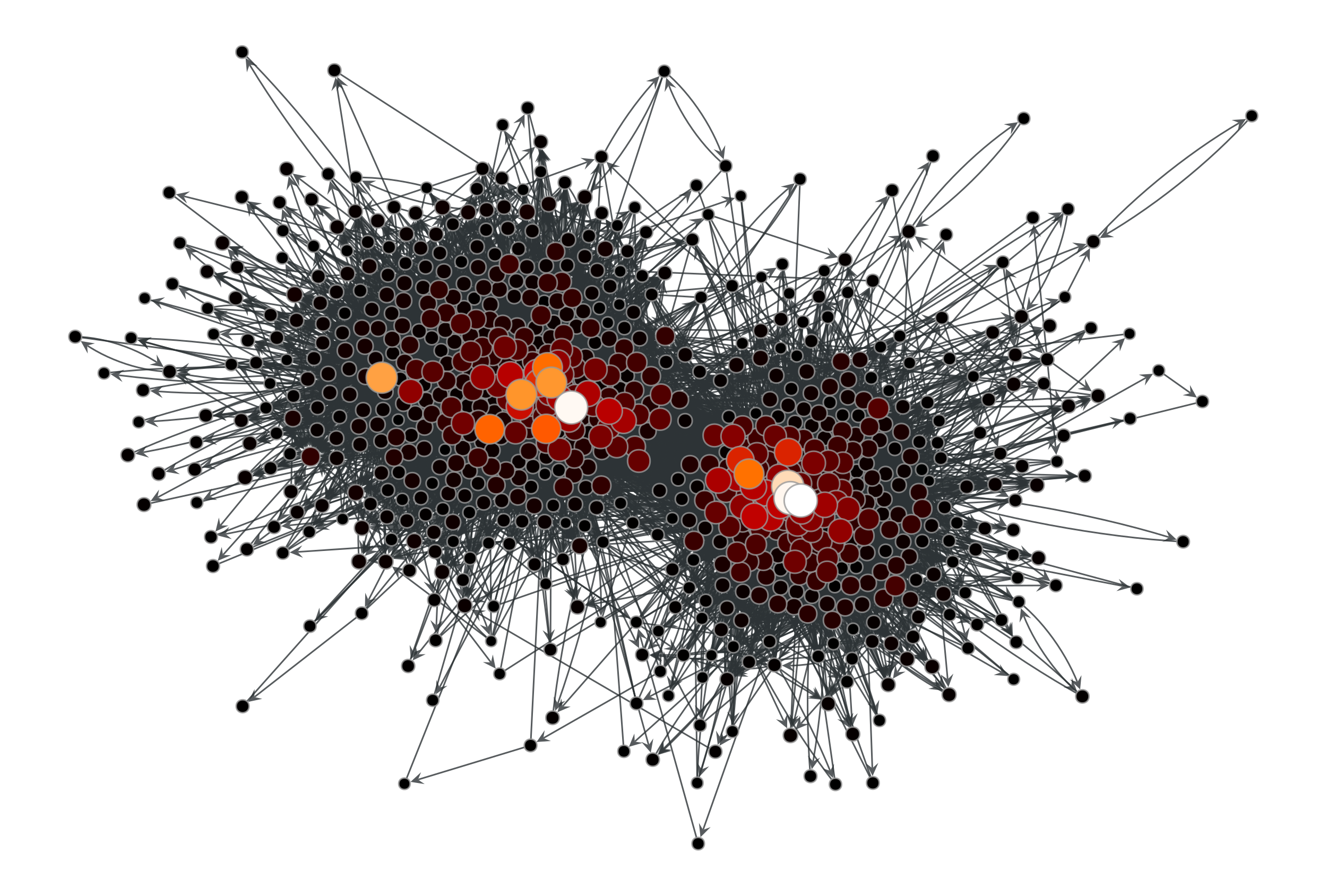# graph_tool.centrality.katz#

graph_tool.centrality.katz(g, alpha=0.01, beta=None, weight=None, vprop=None, epsilon=1e-06, max_iter=None, norm=True)[source]#

Calculate the Katz centrality of each vertex in the graph.

Parameters:
gGraph

Graph to be used.

weightEdgePropertyMap (optional, default: None)

Edge property map with the edge weights.

alphafloat, optional (default: 0.01)

Free parameter $$\alpha$$. This must be smaller than the inverse of the largest eigenvalue of the adjacency matrix.

betaVertexPropertyMap, optional (default: None)

Vertex property map where the local personalization values. If not provided, the global value of 1 will be used.

vpropVertexPropertyMap, optional (default: None)

Vertex property map where the values of eigenvector must be stored. If provided, it will be used uninitialized.

epsilonfloat, optional (default: 1e-6)

Convergence condition. The iteration will stop if the total delta of all vertices are below this value.

max_iterint, optional (default: None)

If supplied, this will limit the total number of iterations.

normbool, optional (default: True)

Whether or not the centrality values should be normalized.

Returns:
centralityVertexPropertyMap

A vertex property map containing the Katz centrality values.

betweenness

betweenness centrality

pagerank

PageRank centrality

eigenvector

eigenvector centrality

hits

authority and hub centralities

trust_transitivity

pervasive trust transitivity

Notes

The Katz centrality $$\mathbf{x}$$ is the solution of the nonhomogeneous linear system

$\mathbf{x} = \alpha\mathbf{A}\mathbf{x} + \mathbf{\beta},$

where $$\mathbf{A}$$ is the (weighted) adjacency matrix and $$\mathbf{\beta}$$ is the personalization vector (if not supplied, $$\mathbf{\beta} = \mathbf{1}$$ is assumed).

The algorithm uses successive iterations of the equation above, which has a topology-dependent convergence complexity.

If enabled during compilation, this algorithm runs in parallel.

References

[katz-new]

L. Katz, “A new status index derived from sociometric analysis”, Psychometrika 18, Number 1, 39-43, 1953, DOI: 10.1007/BF02289026 [sci-hub, @tor]

L. A. Adamic and N. Glance, “The political blogosphere and the 2004 US Election”, in Proceedings of the WWW-2005 Workshop on the Weblogging Ecosystem (2005). DOI: 10.1145/1134271.1134277 [sci-hub, @tor]

Examples

>>> g = gt.collection.data["polblogs"]
>>> g = gt.GraphView(g, vfilt=gt.label_largest_component(g))
>>> w = g.new_edge_property("double")
>>> w.a = np.random.random(len(w.a))
>>> x = gt.katz(g, weight=w)
>>> gt.graph_draw(g, pos=g.vp["pos"], vertex_fill_color=x,
...               vertex_size=gt.prop_to_size(x, mi=5, ma=15),
...               vcmap=matplotlib.cm.gist_heat,
...               vorder=x, output="polblogs_katz.pdf")
<...>Katz centrality values of the a political blogs network of [adamic-polblogs], with random weights attributed to the edges.#